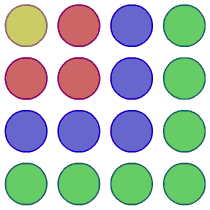You may also likeHave You Got It?

Can you explain the strategy for winning this game with any target?Counting Factors

Is there an efficient way to work out how many factors a large number has?Is There a Theorem?

Draw a square. A second square of the same size slides around the first always maintaining contact and keeping the same orientation. How far does the dot travel?

Picturing Square Numbers

Age 11 to 14Challenge Level

The diagram shows that 1 + 3 + 5 + 7 = 16.
Full Screen InteractivityWhat is the sum of the first $30$ odd numbers?

What is the sum of the first $60$ odd numbers?

Can you describe a method for working these out quickly?

Can you make $3249$ by adding odd numbers in this way?

What is the value of:

$1 + 3 + ... + 149 + 151 + 153$?

$83 + 81+ ... + 5 + 3 + 1$?

$51 + 53 + 55 + ... + 149 + 151 + 153$?

$2 + 4 + ... + 150 + 152 + 154$?

$2 + 6+ ... + 298+ 302+ 306$?

Explain how you worked these out.